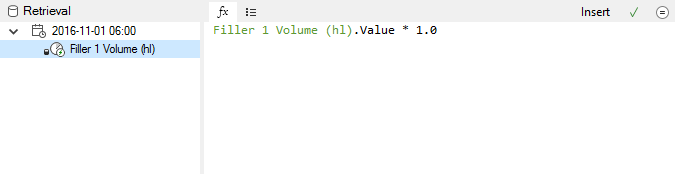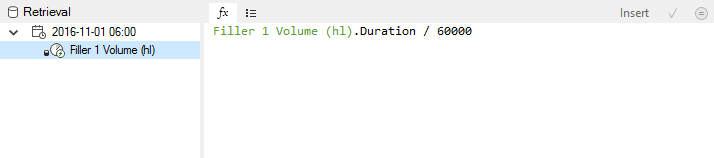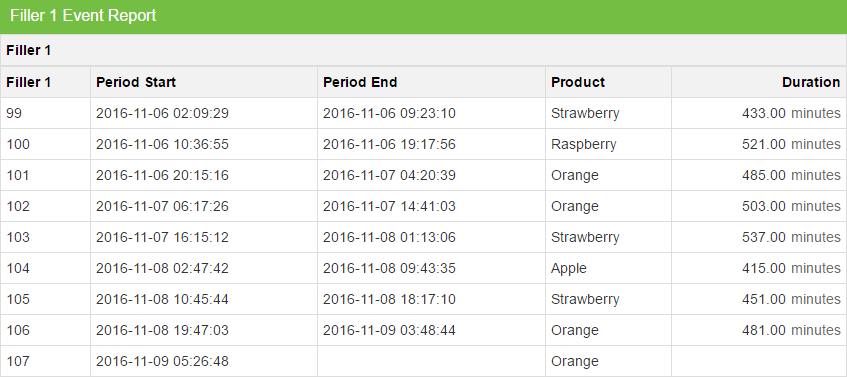### Post

2 followers Follow# Adding a "duration" column to an Event report

You can use a calculated measure to add a "duration" column to an Event report. Let's see how you can do this:

2. Now create a new calculated measure by dragging this measure onto the calculator icon in the "Flow Zone" (see https://support.flow-software.com/hc/en-us/articles/200837342-Create-a-Calculated-Measure)

3. Open the new calculated measure and go to it's "Retrieval" tab. You should see the following default calculation:4. Edit the calculation by changing the .Value to .Duration:The .Duration property provides the number of milliseconds (ms) between the start and the end of a period. This is applicable to both time periods and event periods.

In this example we have divided the duration by 60000 to convert it to minutes.

5. Now you can link this new calculated measure to your Event report definition to provide the following:Flow Support